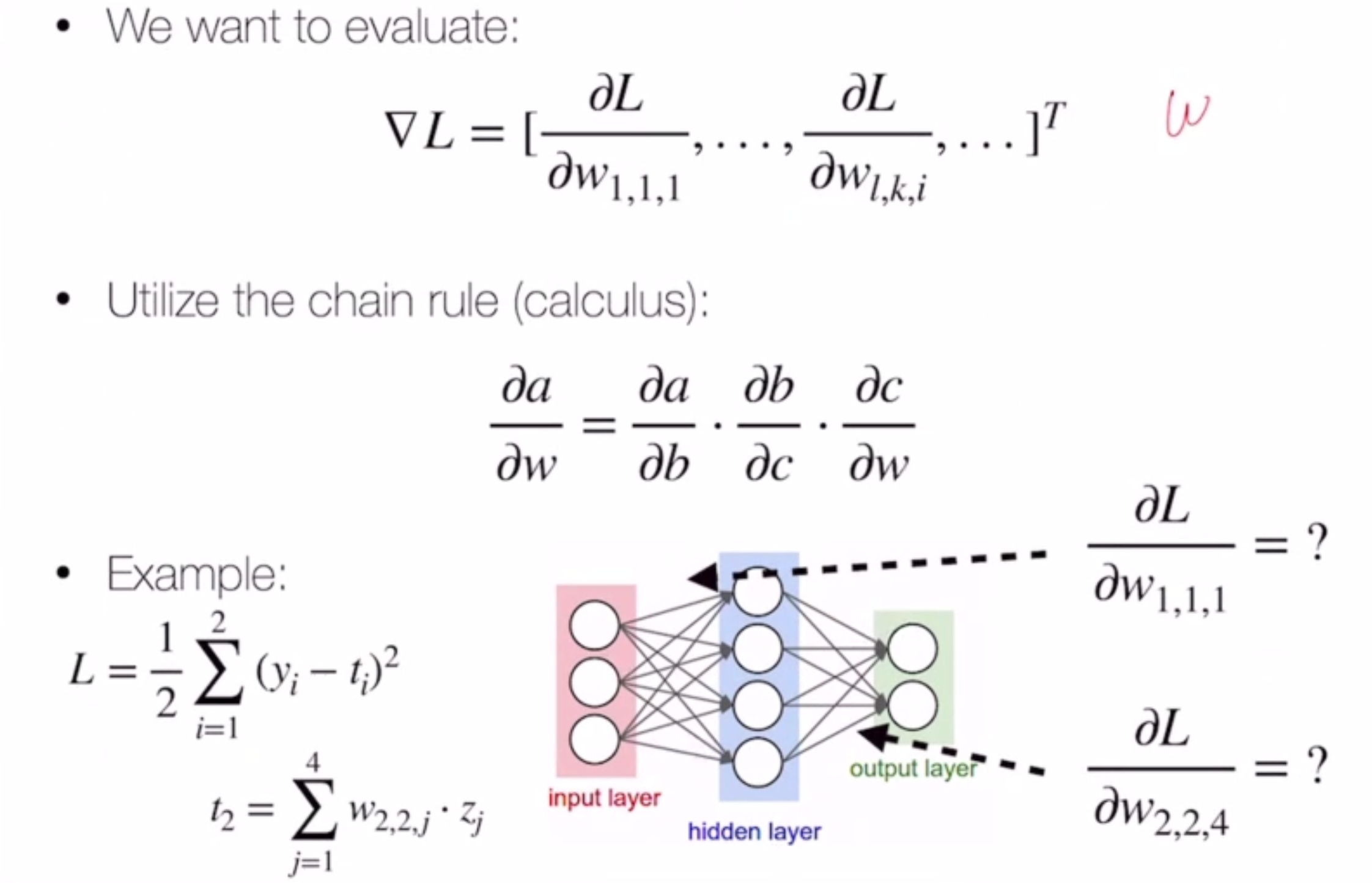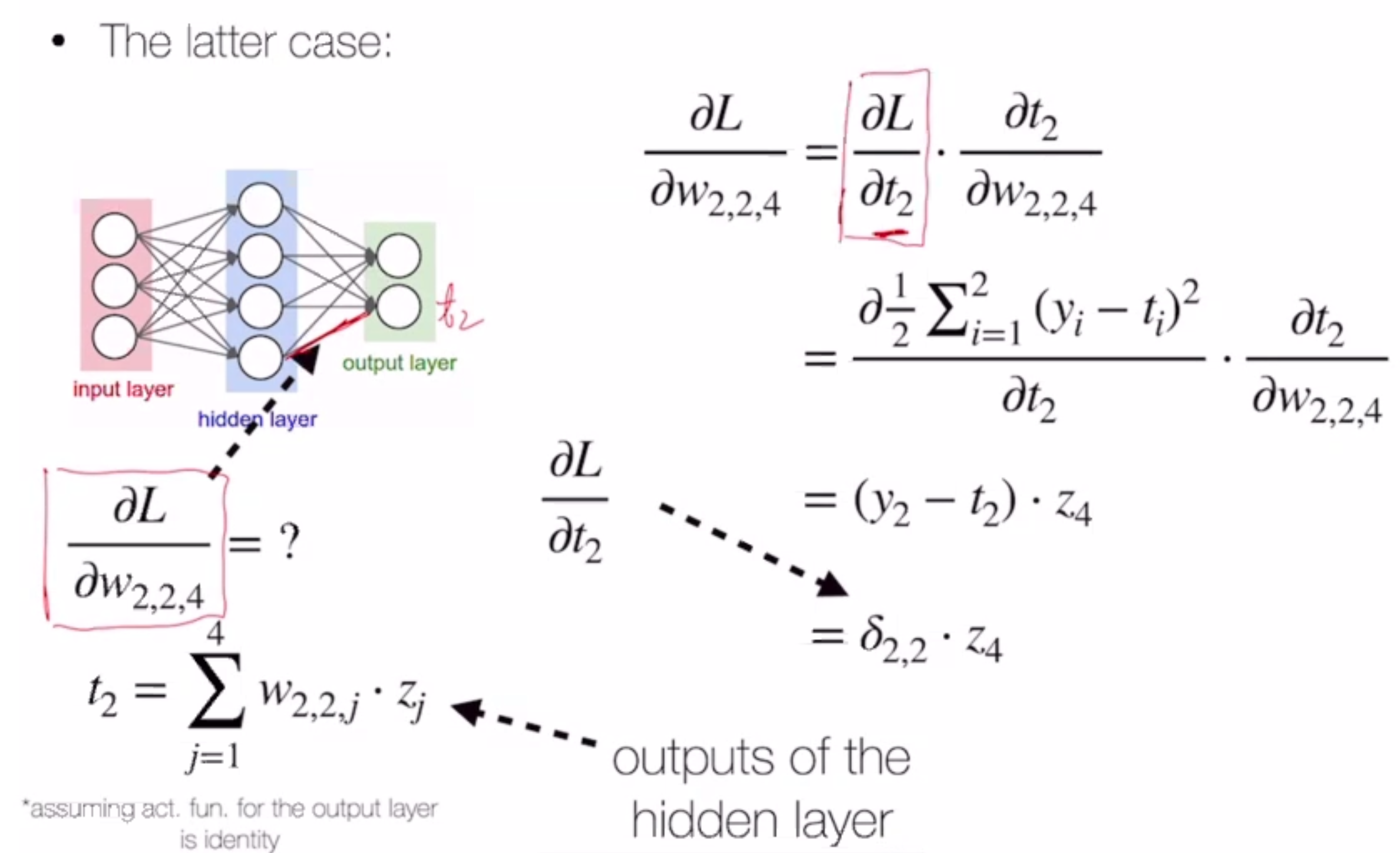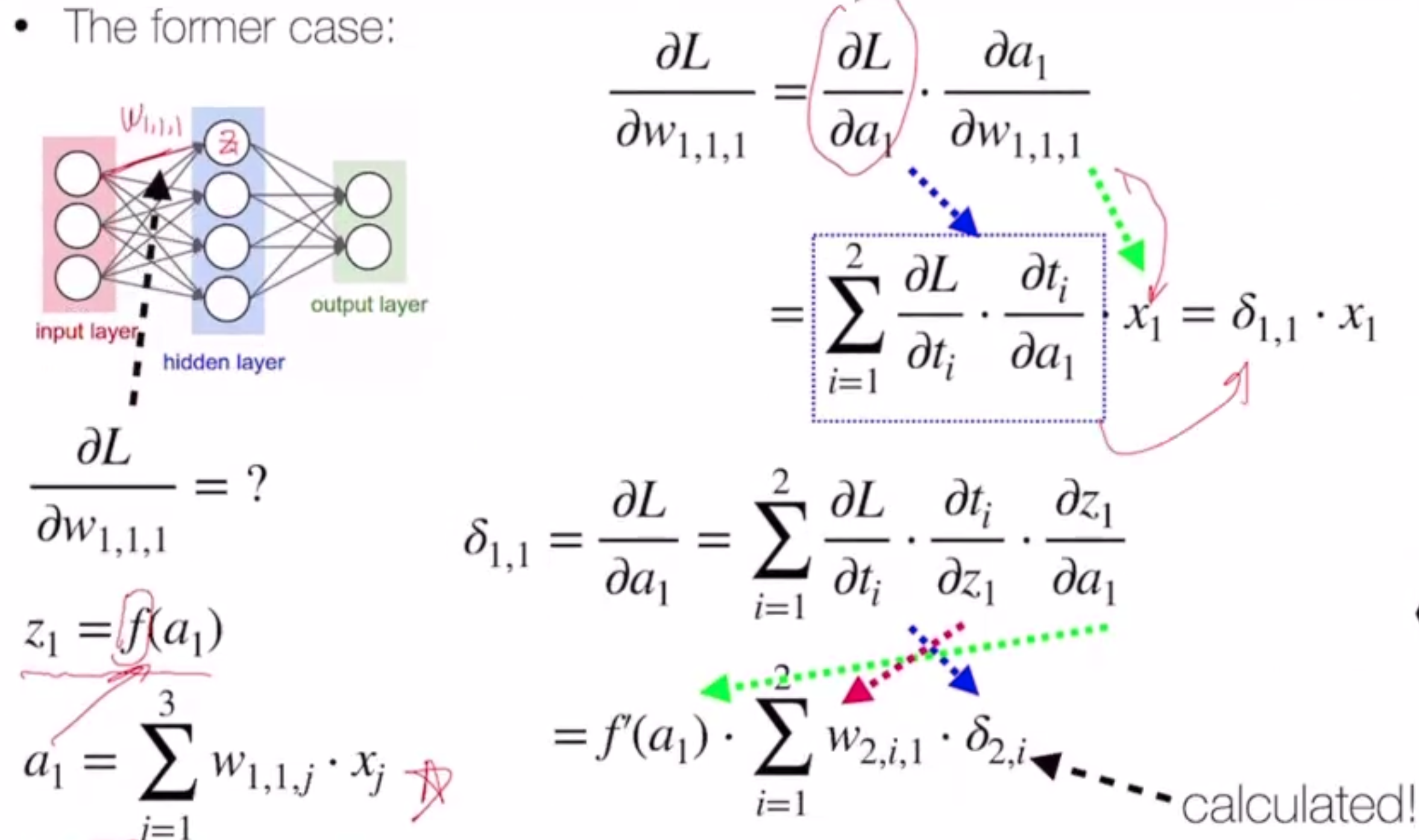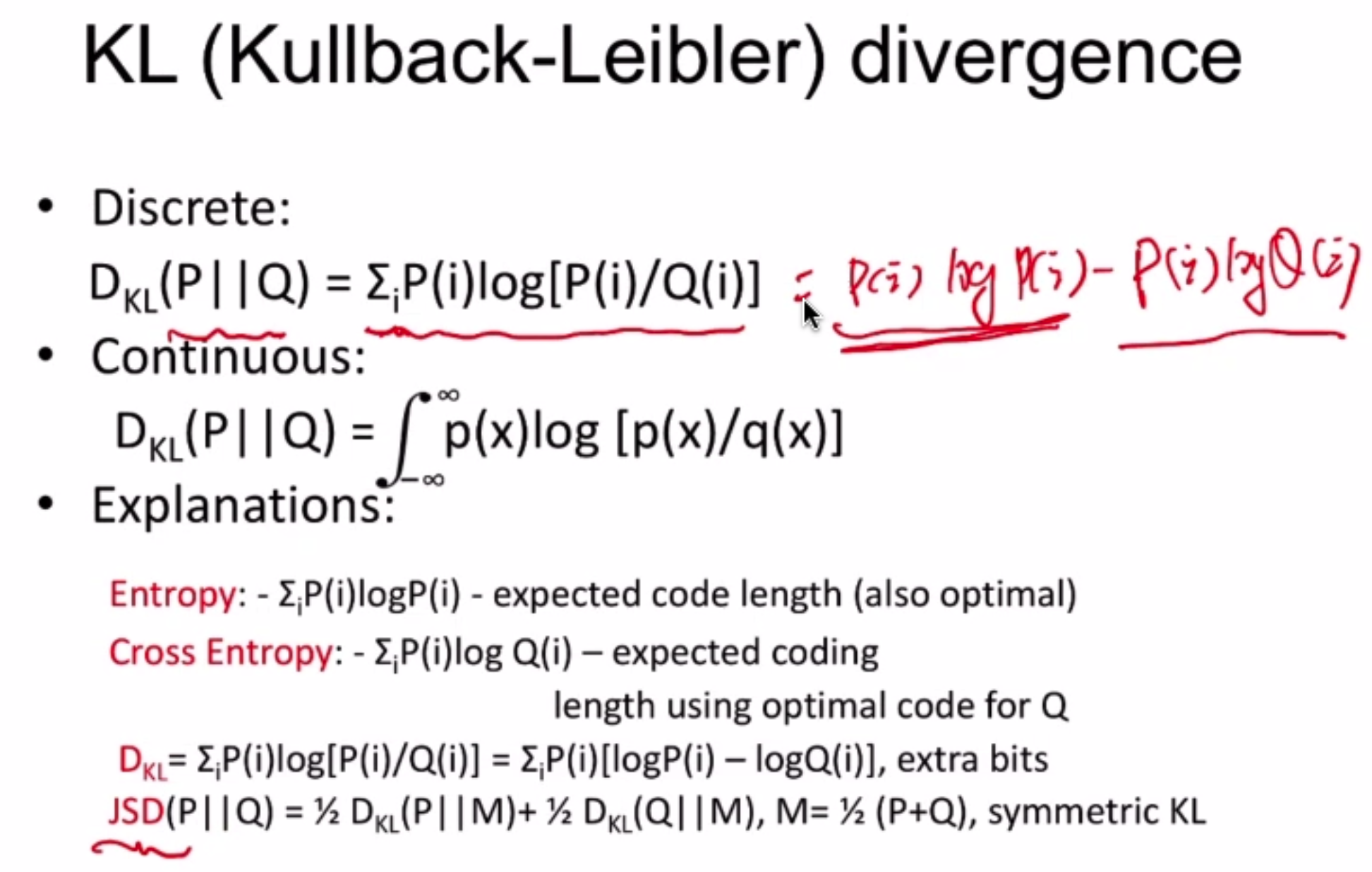# Neural Nets¶

Neural nets can be used to approximate nonlinear functions with a hypothesis!

Neural nets are an intuitive extension of perceptron - think of perceptron as a 1-layer neural net.

But in perceptron, the output is just the sign of the linear combination of the inputs - in NN, we use an activation function

$sign(W_i \cdot x) \to f(W_i \cdot x)$

Activations should be nonlinear - if they’re linear, it’s redundant

## Activations¶

• sign: -1, 0, or 1
• not differentiable
• often used for output layer
• tanh: $$\frac{e^{2x}-1}{e^{2x}+1}$$
• sigmoid: $$\frac{e^x}{1+e^x}$$
• ReLU: $$\max(0, x)$$

## Training¶

Let’s consider the following loss objective on a 2-layer neural net with weights W and v:

$L(w, v) = \min_{W,v} \sum_n \frac{1}{2} (y^n - score)^2$

we just need to find

$\frac{\partial L}{\partial W}, \frac{\partial L}{\partial v}$

We do this using backpropogation.## VAE¶

Whereas normal AE turns images into a latent vector, VAE tries to learn the parameters of a gaussian distribution that the image is a mixture of

$c_i = \exp(\sigma_i)e_i + m_i$

where $$c_i$$ is a component of the latent vector, $$e_i$$ is a random exponential term, and $$m_i$$ and $$\sigma_i$$ are the gaussian variables.

## KL Divergence¶

Roughly, a measure of how close two distributions are to each other (>= 0)## Random¶

Random note: GAN objective can also be written $$\max_D V(G,D) = -2 \log 2 + 2 JSD(P_{data}(x) || P_G(x))$$

### f-GAN¶

Uses a generalized divergence function:

$D_f(q||p) = \int p(x) f[\frac{q(x)}{p(x)}]dx$

by making $$f = \log$$, this is KL divergence